# A Gentle Introduction to Time Series Analysis & Forecasting

What would you do if you knew what the future would look like? That answer might be “buy bitcoin in 2017” for some people. Or for retailers, that answer could be “stocking up on toilet paper or silicon chips in 2019”. Whatever your answer is, we can agree that having information about the future would be advantageous.

Although we have been asking fortune tellers what the future holds for us for a long time, predicting the future is still a challenge. Even with our technology today, the predictions about the weather an hour from now can be massively wrong. However, for some use cases, we can model the behavior of a time series well enough to make quite accurate predictions about the future.

Time series analysis and forecasting are two broad topics that can be overwhelming. Thus, this article will introduce you to the essential concepts of them. To showcase some concepts, we will use code examples in Python and methods from the library statsmodels . Let’s jump in!

### Definitions of Time Series Forecasting and Time Series Analysis

This section will define the three key terms: time series, time series analysis, and time series forecasting.

#### What Is a Time Series?

A time series is a sequence of data points dependent on time. That means that each data point has a timestamp assigned to it. Ideally, these data points are measured at constant intervals (e.g., every day) and in chronological order (e.g., Monday, Tuesday, Wednesday, etc.).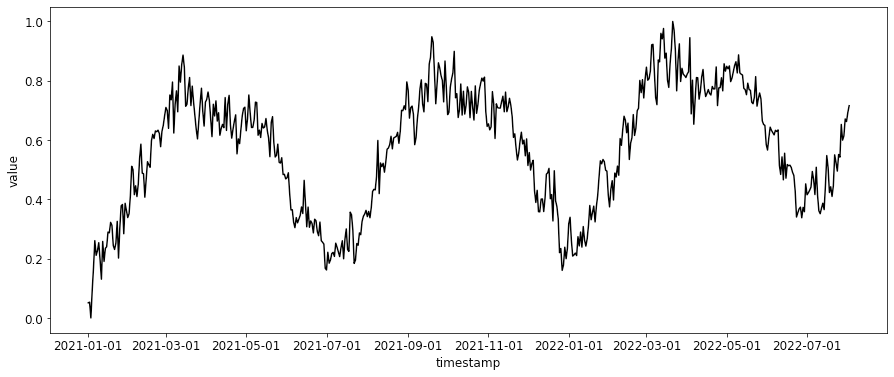Time series are usually numerical values, e.g., sales data, but they can also be categorical, e.g., event data. Time series data usually comes in tabular format (e.g., CSV files) with a column for the timestamps and at least one for the time series values.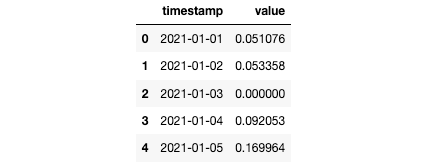#### What Is Time Series Analysis?

Time series analysis is similar to Exploratory Data Analysis (EDA) in the Data Science workflow because it analyzes relationships among variables and outliers [3, 9]. Additionally, we want to find long-term trends or short-term repeating patterns [3, 9].

Similar to standard EDA techniques, plotting and calculating statistical characteristics like mean and standard deviation are also essential for time series analysis . However, you must use additional methods to explore the added dimension of time (see Fundamentals of Time Series Analysis).On the one hand, time series analysis can be a standalone concept. On the other hand, it can be an EDA step during the time series forecasting workflow because the insights you gain about the time series and its pattern can help you determine which model to use .

#### What Is Time Series Forecasting?

In time series forecasting, we try to predict how a sequence of observations will continue in the future. For this purpose, we first analyze historical data similar to time series analysis. Then we fit a model to the historical data to make our predictions.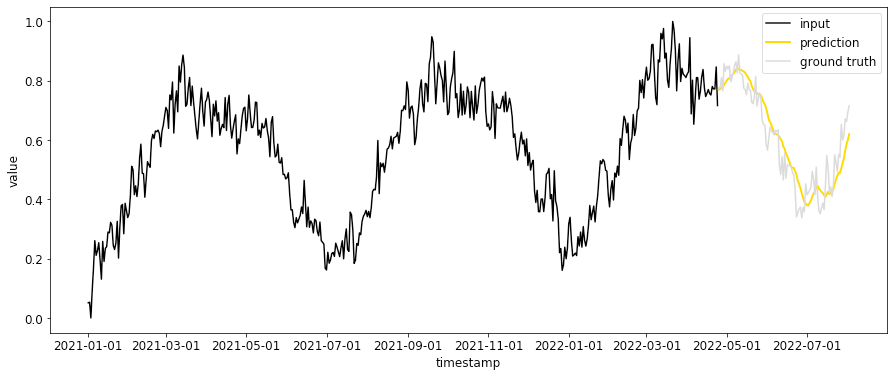Time series forecasting has a wide variety of problem settings. They can differ in the following aspects:
• number of observed time series to predict (univariate vs. multivariate)
• prediction time frame (short-term vs. long-term)

### Use Cases for Time Series Analysis and Forecasting

Time series analysis and time series forecasting have various use cases. Here are just a few examples:
• Finance:  One of the most popular examples of time series forecasting is stock price predictions. While we all would like to know how to calculate which stock will be the next Google or Amazon, this is also one of the most challenging use cases of time series forecasting.
• Weather:  One of the other commonly known use case of time series forecasting is weather forecasting. It also happens to be an excellent example to showcase that forecasts with a shorter prediction horizon (e.g., tomorrow’s weather) are usually more reliable than forecasts with a longer prediction horizon (e.g., the weather a week from today).
• Demand:  Pretty much anything with demand is a good use case for time series analysis and forecasting. Examples include power generation, store inventory, staffing, the number of passengers on a bus or flight, or traffic on a highway.
As you can likely read between the lines, some types of time series are easier to forecast than others. This depends on the following factors :
• How well do we understand the influencing factors?
• How much data do we have available?

### Fundamentals of Time Series Analysis

This section will introduce you to some of the essential concepts of time series analysis: The components of a time series and stationarity.

#### Components of a Time Series

When choosing a forecasting method, we will first need to identify the patterns in the time series data and then select a model that can capture the patterns adequately . An observed time series can be decomposed into the following five components:
• Level : the average value.
• Trend: a long-term increasing or decreasing tendency.
• Seasonality:  a short-term repeating pattern at fixed periods, e.g., weekly, monthly, or annually.
• Cycle:  a short-term repeating pattern (not at fixed periods).
• Noise:  irregular or even random, short-term fluctuations.
Seasonal and cyclic patterns can be challenging to distinguish in time series data. If the frequency of a reoccurring pattern is constant regarding the calendar, then the pattern is seasonal. Otherwise, it is cyclic .

We can use the function seasonal_decompose() from the statsmodels  library. It is a naive decomposition method and decomposes a time series into only three components: trend, seasonality, and noise (residual) .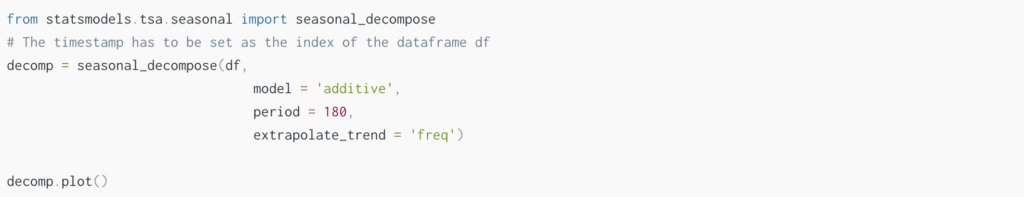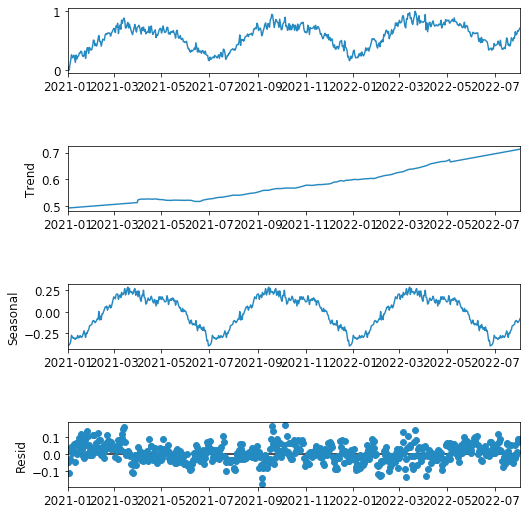#### What Is Stationarity and Why Is It Important for Time Series Analysis?

Stationarity describes the concept that how a time series is changing will remain the same in the future . In mathematical terms, a time series is stationary when its statistical properties (mean, variance, and covariance) are independent of time .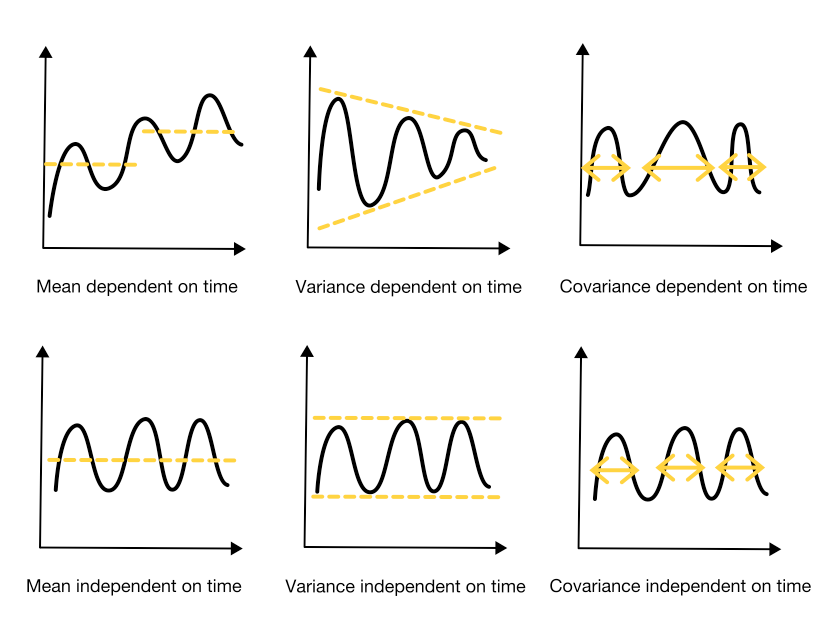When the future is similar to the past, it is easier to forecast . Thus, a stationary time series is easier to model than a non-stationary one because its statistical properties will remain the same. Therefore, a lot of time series forecasting models assume stationarity.

If a time series is non-stationary, you can try to make it stationary by, e.g., differencing it.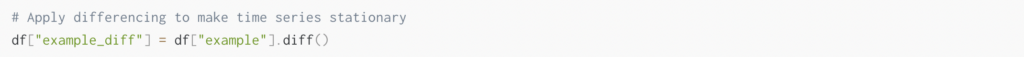### Fundamentals of Time Series Forecasting

This section will discuss popular time series forecasting models and how to train and validate them.
You can approach time series forecasting with a wide variety of models. Forecasting methods range from simple ,  like using the last observation as the prediction - over classical, to highly complex ML models .

Naive approach: In a naive forecast, the predicted value is simply the value of the most recent observation. This basic method is often used as a benchmark to evaluate the performance of more sophisticated models.Thus, as a first step when you track your experiments with Weights and Biases, creating a baseline with a naive approach to test your experiments against would be recommended.💡

Regression-based time series forecasting: Regression-based time series forecasting methods assume that the time series has a linear relationship with other time series . This assumption about the linear relationship results in a model of a trend but not of seasonality.

Exponential smoothing: Exponential smoothing was proposed in the late 1950s and is a classical forecasting method [1, 2, 13]. Its popularity results from the fact that this method generates reliable (short-term) forecasts quickly while requiring relatively little memory space .

This forecasting method uses weighted averages of past observations. Taking the averages over all past observations reduces the noise of the time series (smoothing), and the weights decay exponentially as the observations get older .

Autoregressive Integrated Moving Average (ARIMA): ARIMA is a class of forecasting methods that models a time series based on its autocorrelations [3, 9]. The models are a combination of the following building blocks:
• Autoregressive (AR): makes predictions based on a linear combination of its past values.
• Moving Average (MA): makes predictions based on a linear combination of past forecasts’ errors.
Prophet: Prophet is an open-source time series framework designed to work out of the box and developed by Facebook’s Core Data Science team . The main idea is based on the assumption that the time series can be decomposed into four components: trend, seasonal component (e.g., weekly or yearly), deterministic irregular component (e.g., holidays), and noise.

Machine Learning:  In recent years, Machine Learning models have gained popularity for time series forecasting. In the 2020 Makridakis competition (M5 competition) , which is a series of competitions to evaluate and compare different time series forecasting methods, the best predictions were produced by Neural Networks or Gradient Boosting frameworks [4, 5, 6].

#### Training and Validating Time Series Forecasting Models

For time series forecasting, you can’t apply the same cross-validation strategies as for regular Machine Learning models because of the time aspect. Thus, the training data must be older than the validation data for training and validating forecasting models.
For cross-validation, you can use the Time Series Split library .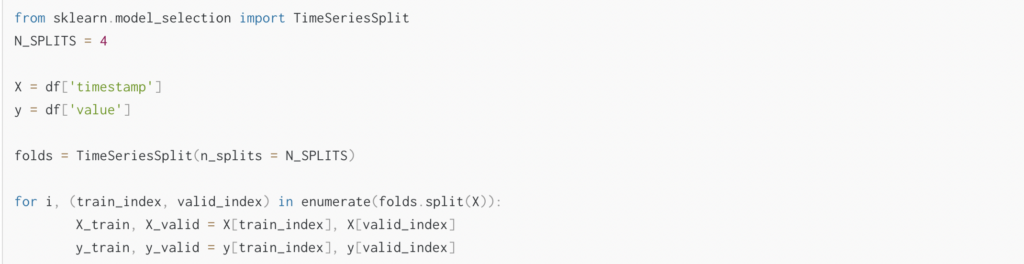In addition to considering the time aspect, you can decide whether to have a fixed length of input data (sliding window) or an extending length of input data (expanding window) for training.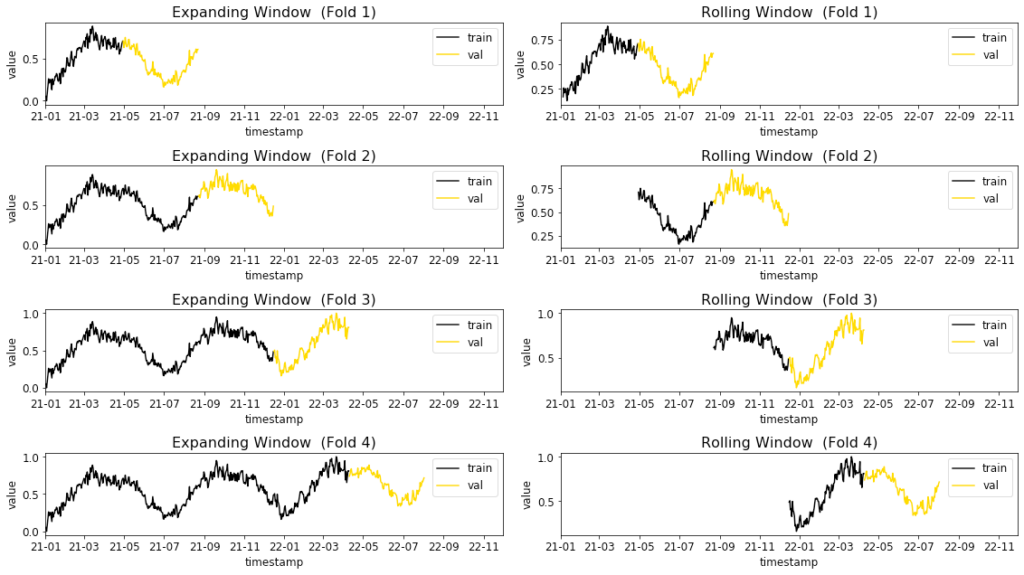### Conclusion

Time series analysis and time series forecasting are two broad topics and can be intimidating to beginners. Thus, this article aims to give you a gentle introduction to some essential concepts.

We first learned that time series analysis is a descriptive approach. Similarly to EDA in the Data Science workflow, time series analysis aims to find patterns and relationships in the data. But, the added dimension of time requires additional analysis aspects.
You learned that a time series could have five components: level, trend, seasonality, cycle, and noise. These components indicate different relationships between the time series values and the timestamps. Also, you learned how to check whether the way a time series is changing will stay the same in the future (stationarity).

In contrast to time series analysis, time series forecasting makes predictions based on the patterns in the time series. We discussed that some forecasting use cases are easier to predict than others.

We also discussed a small selection of time series forecasting methods ranging from naive to classical (regression, ARIMA, exponential smoothing) to modern (prophet, neural networks, gradient boosted frameworks) approaches. Also, you learned that you have to consider the time aspect during training and validation of time series forecasting models.